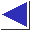# comp.lang.c FAQ list ·Question 6.3

Q: So what is meant by the ``equivalence of pointers and arrays'' in C?

A: Much of the confusion surrounding arrays and pointers in C can be traced to a misunderstanding of this statement. Saying that arrays and pointers are ``equivalent'' means neither that they are identical nor even interchangeable. What it means is that array and pointer arithmetic is defined such that a pointer can be conveniently used to access an array or to simulate an array. In other words, as Wayne Throop has put it, it's ``pointer arithmetic and array indexing [that] are equivalent in C, pointers and arrays are different.'')

Specifically, the cornerstone of the equivalence is this key definition:

A reference to an object of type array-of-T which appears in an expression decays (with three exceptions) into a pointer to its first element; the type of the resultant pointer is pointer-to-T.

That is, whenever an array appears in an expression, the compiler implicitly generates a pointer to the array's first element, just as if the programmer had written &a. (The exceptions are when the array is the operand of a sizeof or & operator, or is a string literal initializer for a character array. [footnote] See questions 6.23, 6.12, and 1.32, respectively.)

As a consequence of this definition, and in spite of the fact that the underlying arrays and pointers are quite different, the compiler doesn't apply the array subscripting operator [] that differently to arrays and pointers, after all.[footnote] Given an array a and pointer p, an expression of the form a[i] causes the array to decay into a pointer, following the rule above, and then to be subscripted just as would be a pointer variable in the expression p[i] (although the eventual memory accesses will be different, as explained in question 6.2). If you were to assign the array's address to the pointer:

```	p = a;
```
then p and a would access the same element.

This harmony of access explains how pointers can access arrays, serve in their stead as function parameters (see question 6.4), and simulate dynamic arrays (see question 6.14).